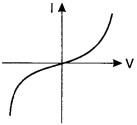# Physics class 12 chapter 3 Objective Questions

## Current Electricity Objective Questions

1. An electric heater is connected to the voltage supply. After few seconds, current gets its steady value then its initial current will be

(a) equal to its steady current

(b) slightly higher than its steady current

(c) slightly less than its steady current

(d) zero

Answer: (b) slightly higher than its steady current

2. In the series combination of two or more than two resistances

(a) the current through each resistance is same.

(b) the voltage through each resistance is same.

(c) neither current nor voltage through each re-sistance is same.

(d) both current and voltage through each resis¬tance are same.

Answer: (a) the current through each resistance is same.

3. Combine three resistors 5 Q, 4.5 Q and 3 Q in such a way that the total resistance of this combination is maximum

(a) 12.5 Q

(b) 13.5 Q

(c) 14.5 Q

(d) 16.5 Q

Answer: (a) 12.5 Q

4. In parallel combination of n cells, we obtain

(a) more voltage

(b) more current

(c) less voltage

(d) less current

Answer: (b) more current

5. In a Wheatstone bridge if the battery and galvanometer are interchanged then the deflection in galvanometer will

(a) change in previous direction

(b) not change

(c) change in opposite direction

(d) none of these.

Answer: (b) not change

6. When a metal conductor connected to left gap of a meter bridge is heated, the balancing point

(a) shifts towards right

(b) shifts towards left

(c) remains unchanged

(d) remains at zero

Answer: (a) shifts towards right

7. In a potentiometer of 10 wires, the balance point is obtained on the 7th wire. To shift the balance point to 9th wire, we should

(a) decrease resistance in the main circuit.

(b) increase resistance in the main circuit.

(c) decrease resistance in series with the cell whose emf is to be measured.

(d) increase resistance in series with the cell whose emf is to be determined.

Answer: (d) increase resistance in series with the cell whose emf is to be determined.

8. AB is a wire of potentiometer with the increase in the value of resistance R, the shift in the balance point J will be

(a) towards B

(b) towards A

(c) remains constant

(d) first towards B then back towards A.

Answer: (a) towards B

9. A charge is moving across a junction, then

(a) momentum will be conserved.

(b) momentum will not be conserved.

(c) at some places momenturii will be conserved and at some other places momentum will not be conserved.

(d) none of these.

Answer: (d) none of these.

10. The I-V characteristics shown in figure represents

(a) ohmic conductors

(b) non-ohmic conductors

(c) insulators

(d) superconductors

11. Which of the following is correct for V-I graph of a good conductor?(a) ohmic conductors

(b) non-ohmic conductors

(c) insulators

(d) superconductors

Answer: (b) non-ohmic conductors

12. The resistivity of alloy manganin is

(a) Nearly independent of temperature

(b) Increases rapidly with increase in temperature

(c) Decreases with increase in temperature

(d) Increases rapidly with decrease in temperature

Answer: (a) Nearly independent of temperature

physics class 12 chapter 3 english medium, current electricity class 12, current electricity class 12 ncert solutions, physics class 12 chapter 3 in english, physics class 12th chapter 3, physics chapter 3 class 12 important questions in english, important questions for class 12 physics 2022, physics important questions class 12, cbse class 12 physics chapter 3 important questions, physics important questions class 12 chapter 3, current electricity objective question in english, objective questions on current electricity for class 12, current electricity class 12 objective questions pdf, 12th physics chapter 3 in english, class 12th physics chapter 3 mcq, class 12 physics chapter 3 current electricity, class 12 physics chapter 3 ncert pdf, physics class 12 chapter 3 ncert solutions, class 12 physics chapter 3 board questions, cbse class 12 physics chapter 3 ncert solutions, cbse class 12 physics chapter 3 important questions, ncert solutions for class 12 physics chapter 3 pdf, physics 12 chapter 3, physics class 12 ncert solutions chapter 3 pdf, science sangrah official, physics, 12th physics, class 12 physics chapter 3 in english, bhautiki kaksha 12, 12th physics important questions in english, board exam 2022,